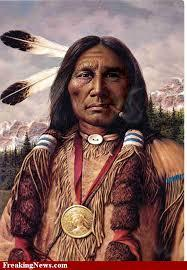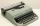# Ethnicity

Share of ethnicity XY is 26%, which is 1/9 more than in the prewar period.

What was the share of that ethnicity in the pre-war period?

Correct result:

p =  23.4 %

#### Solution:

$26/100 \cdot x = (1+1/9)p/100 \cdot x \ \\ 26= (1+1/9)p \ \\ p = \dfrac{ 26 }{ 1+1/9} \ \\ p = 23.4 \%$We would be very happy if you find an error in the example, spelling mistakes, or inaccuracies, and please send it to us. We thank you!Tips to related online calculators
Do you have a linear equation or system of equations and looking for its solution? Or do you have quadratic equation?

## Next similar math problems:

• Base, percents, valueBase is 344084 which is 100 %. How many percent is 384177?
• Apples 2James has 13 apples. He has 30 percent more apples than Sam. How many apples has Sam?
• NumberWhat number is 20 % smaller than the number 198?
• ClassIn 7.C clss are 10 girls and 20 boys. Yesterday was missing 20% of girls and 50% boys. What percentage of students missing?
• New refrigeratorNew refrigerator sells for 1024 USD, Monday will be 25% discount. How much USD will save, and what will be the price?
• Percents - easyHow many percent is 432 out of 434?
• IronIron ore contains 57% iron. How much ore is needed to produce 20 tons of iron?
• AssistantAssistant rewrote 15% of the entire manuscript in 12 hours. How many hours must still write to rewrite the rest of the manuscript?
• Sales offGoods is worth € 70 and the price of goods fell two weeks in a row by 10%. How many % decreased overall?
• Seeds 2How many seeds germinated from 1000 pcs, when 23% no emergence?
• Conference148 is the total number of employees. The conference was attended by 22 employees. How much is it in percent?
• Unknown numberIdentify unknown number which 1/5 is 40 greater than one tenth of that number.
• Percentage - fractionsAbout what percentage we must increase number 1/6 to get number 1/3?
• EquationSolve the equation: 1/2-2/8 = 1/10; Write the result as a decimal number.
• GivenGiven 2x =0.125 find the value of x
• Simplify 2Simplify expression: 5ab-7+3ba-9
• Simple equationSolve for x: 3(x + 2) = x - 18